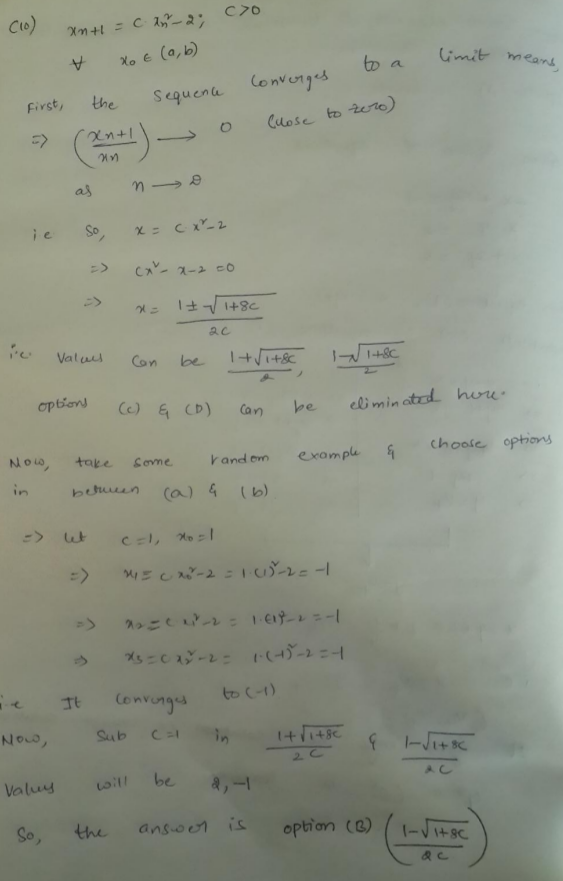GFG App
Open AppBrowser
Continue

# GATE | Gate IT 2007 | Question 74

Consider the sequence <xn>, n>= 0 defined by the recurrence relation xn + 1 = c . xn2– 2, where c > 0.

Suppose there exists a non-empty, open interval (a, b) such that for all x0 satisfying a < x0 < b, the sequence converges to a limit. The sequence converges to the value?
(A) (1 + (1 + 8c)1/2)/2c
(B) (1 – (1 + 8c)1/2)/2c
(C) 2
(D) 2/(2c-1)